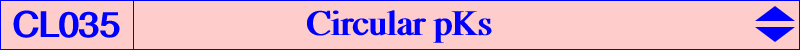Let P = p : q : r be a fixed point and M a variable point. Denote by AmBmCm the pedal triangle of M and by MaMbMc the triangle formed by the orthogonal projections of M on the cevian lines of P. If P = H, these two triangles are always perspective and the perspector is f(P), equivalently the midpoint of the points M, H/M (H-Ceva conjugate of M) or the barycentric product of M and its orthocorrespondent. If P is distinct of H, the triangles are perspective if and only if M lies on a circular pK with pivot P whose pole is g(P). The first barycentric coordinate of g(P) is : a^2[-2 SA p^2 + a^2(qr+rp+pq) - p(b^2q+c^2r)]. g(P) is the pole of the isoconjugation which swaps P and igP, the inverse (in the circumcircle) of the isogonal conjugate of P.Circular isogonal pKs g maps any real point at infinity to the Lemoine point K hence there are infinitely many isogonal circular pKs. The most famous is the Neuberg cubic K001 with P = X(30) = infinite point of the Euler line. See examples in the table below and also Special Isocubics §4.1.1.P centers on the cubic cubic X30 X1, X3, X4, X13, X14, X15, X16, X30, X74, X370, X399, X484, X616, X617, X1138, X1157, X1263, X1276, X1277, X1337, X1338, X2132, X2133, etc K001 X512 X1, X99, X512, X2142, X2143, Brocard points K021 X513 X1, X100, X513, X1054 K661 X515 X1, X36, X40, X80, X84, X102, X515 K269 X518 X1, X105, X518, X1282 X522 X1, X109, X522, X1768 X523 X1, X110, X523, X21381 K1155 X524 X1, X2, X6, X111, X524 K1156 X527 X1, X9, X57, X527, X2291 X698 X1, X194, X698, X699 X736 X1, X32, X76, X736, X737 X744 X1, X31, X75, X744, X745 X1503 X1, X20, X64, X147, X1297, X1503 K270Circular pKs with pivot H The reciprocal image of H under g is the orthic axis. Hence, any circular pK with pole on the orthic axis must have its pivot at H and then, the singular focus is the antipode of the red point on the nine-point circle. The following table gives examples of such cubics. See also CL019.Pole centers on the cubic cubic X230 X4, X487, X488 X232 X4, X114, X371, X372, X511, X2009, X2010 K337 X468 X2, X4, X126, X193, X468, X524, X671, X2374 K209 X523 X4, X127, X525, X1289 X647 X4, X122, X520, X1301 X650 X4, X123, X521 X1886 X4, X118, X516, X917 X1990 X4, X13, X14, X30, X113, X1300 K059 = O(X4) X2489 X4, X112, X115, X512 X2501 X4, X107, X125, X523 X3003 X3, X4, X131, X155, X265, X403, X1299, X1986 K339Circular pKs with pole W ≠ K and pivot P ≠ H For any pole W = p : q : r different of K, there is one and only one circular pK with pole W. Its pivot is P = h(W) where h is the reciprocal transformation of g. The first barycentric coordinate of P is : b^2c^2p(q+r-p) - (b^4pr+c^4rp-a^4qr). See Special Isocubics §4.2.1. Recall that, for any pivot P = u : v : w different of H, there is one and only one circular pK with pivot P. See Table 12 for a selection of such cubics with given pivot P. Any cubic of this type is actually an isogonal pK with respect to a triangle A2B2C2 where A2 is the intersection of the lines A, gcP and Pa, P/inf where gcP is the isogonal conjugate of the complement of P (a point on the circum-conic with perspector W and on the cubic), PaPbPc is the cevian triangle of P, P/inf is the P-Ceva conjugate of the real infinite point of the cubic. Obviously, inf is the pivot of the cubic when the reference triangle is A2B2C2. See the Droussent cubic K008 for an example of such triangle A2B2C2.Circular pKs passing through a given point A circular pK with pivot P contains • the incenter X1 if and only if P lies on the line at infinity (isogonal pKs, see above) or on the Feuerbach hyperbola. In this latter case, the pole lies on K1050 = nK(X32, X513, X6) = cK(#X6, X513). • the centroid X2 if and only if P lies on the Droussent cubic K008. The pole lies on K043. • the circumcenter X3 if and only if P lies on the circumcircle (see inversible pKs) or on the Euler line. In this latter case, the pole W must lie on the Brocard axis OK and the isoconjugate P* of the pivot is the inverse of the isogonal conjugate of P, a point on the Jerabek strophoid K039. The construction is easy since we know P and P* (see Special Isocubics §1.4.3 and §4.2.1). The inversive image of this cubic in the circumcircle is another cubic of the same type with pivot the inverse of P in the circumcircle and pole the conjugate (on the line OK) of W in the circum-conic with perspector X(184). For example, with P = X(30) we obtain K001 and K073, with P = X(2) we find K043 and K108. • the orthocenter X4 if and only if P lies on the Ehrmann strophoid K025. More precisely, if P is H, the pole lies on the line at infinity (see CL019). If P is not H, the pole lies on the circum-conic passing through G and K. For example, with P = G, we obtain the Droussent cubic K008. • the Lemoine point X6 if and only if P lies on K273 = pK(X111, X671). The isopivot lies on K108 and the pole lies on K1048 = pK(X32 x X111, X111), the barycentric product X(111) x K043. • the Fermat points X13, X14 if and only if P lies on Kn = K060. The pole lies on K095. • the isodynamic points X15, X16 if and only if P lies on the Neuberg cubic K001. The isopivot lies on K073 and the pole lies on K1049 = pK(X6 x X50, X6), the barycentric product X(6) x K856. *** More generally, a circular pK with pivot P passes through a given point Q if and only if P lies on a circular circum-cubic K_Q with equation ∑ a^2 v w (w y - v z) [-a^2 (x+y) (x+z) + b^2 x (x+y) + c^2 x (x+z)] = 0 which contains H, Q, agQ, giQ, the infinite point ∞Q of Q-gQ, the Ceva point CQ of Q and iQ (on the circumcircle) and also the points S, T, X, Y such that: S = H-giQ /\ Q-CQ, T = Q-giQ /\ agQ-CQ, X second intersection of the line Q-gQ with the rectangular circum-hyperbola passing through gQ and giQ, isogonal transform of the line OQ, Y second intersection of the line H-∞Q with the rectangular circum-hyperbola passing through CQ. The pole also lies on a circum-cubic Ω_Q passing through K, Q x igQ and more generally the poles of all the pKs with pivots given above. Furthermore, K_Q has a flex at Q if and only if Q lies on K028, the Musselman (third) cubic. the polar conics of X(5) and ∞Q in K_Q are always rectangular hyperbolas. In other words, the orthic line of K_Q (and K_gQ) is the line X(5)-∞Q. thus, the polar conic of Q in K_Q is a rectangular hyperbola if and only if Q lies on K005, the Napoleon cubic. In this case, K_Q is a focal cubic. For example, with Q = X(4), X(54) we find K025, K466 respectively. K_Q is a K0 if and only if Q lies on K018, the Brocard (second) cubic, and in this case, it is a pK. For example, with Q = X(111), X(524) we find K273, K008 respectively. *** When Q lies on the circumcircle, K_Q is psK(Q, tgQ, H) therefore it is tangent at A, B, C to the symmedians. Hence, all these cubics form a pencil and the singular focus lies on the circle C(O, 2R). K_Q meets the sidelines of ABC at the vertices of the cevian triangle of tgQ, the isotomic conjugate of the isogonal conjugate of Q, a point on the Steiner ellipse. The tangent at Q passes through O. The infinite real point of K_Q is the isogonal conjugate of Q and the real asymptote envelopes a deltoid, the homothetic of the Steiner deltoid under h(G,4). See the figure and the table below.Q points on the cubic K_Q cubic remark X(74) X(3), X(4), X(30), X(74) K447 X(98) X(4), X(76), X(98), X(511) X(104) X(4), X(8), X(104), X(517) X(106) X(1), X(4), X(106), X(519), X(1168), X(1320) X(107) X(4), X(107), X(250), X(520) X(108) X(4), X(59), X(108), X(521) X(111) X(2), X(4), X(6), X(23), X(111), X(524), X(671), X(895) K273 pK X(112) X(4), X(112), X(249), X(525) X(733) X(4), X(83), X(732), X(733), X(1916) X(759) X(4), X(21), X(58), X(80), X(758), X(759), X(1325) K306 X(1141) X(4), X(54), X(265), X(1141), X(1154) X(2291) X(4), X(9), X(57), X(527), X(1156), X(2291) X(2373) X(4), X(69), X(316), X(1177), X(2373), X(2393) X(2380) X(4), X(14), X(15), X(532), X(1337), X(2380) X(2381) X(4), X(13), X(16), X(533), X(1338), X(2381)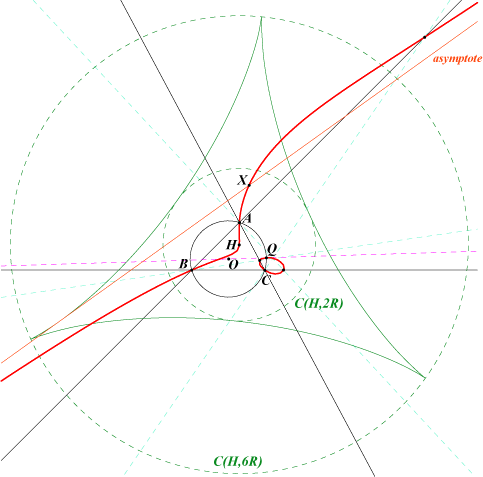*** When Q lies on the line at infinity, K_Q contains the vertices Ga, Gb, Gc of the antimedial triangle. Hence, all these cubics form a pencil and the singular focus lies on the circle C(H, R).Q points on the cubic K_Q cubic remark X(30) X(4), X(20), X(30), X(146), X(265), X(1138), X(1294) K449 X(511) X(4), X(147), X(315), X(511), X(1297), X(1670), X(1671), X(1916) K1131 X(514) X(4), X(150), X(514), X(1305) X(517) X(4), X(153), X(517), X(1295), X(1320) X(519) X(4), X(8), X(80), X(519), X(2370) X(523) X(4), X(523), X(925) focus X(265) X(524) X(2), X(4), X(67), X(69), X(316), X(524), X(671), X(858), X(2373) K008 pK X(527) X(4), X(7), X(329), X(527) X(532) X(4), X(13), X(532), X(616), X(622) X(533) X(4), X(14), X(533), X(617), X(621) X(1154) X(3), X(4), X(1154), X(3153) X(2393) X(4), X(23), X(66), X(895), X(1370), X(2393)*** K_Q and K_gQ generate a pencil of circular cubics passing through H and meeting the line at infinity at the same real point S, namely that of the line Q-gQ. This pencil contains : • pK(H x S, H) where H x S is a point on the orthic axis, see above, • the decomposed cubic which is the union of the line at infinity and the rectangular circum-hyperbola H_Q passing through the isogonal conjugate of the NK-Transform of Q. Recall that the NK-Transform of Q is the pole of the line Q-gQ in the circum-conic passing through Q and gQ. It follows that all these cubics must have two other common points. These points are the intersections of the polar of S in H_Q with H_Q.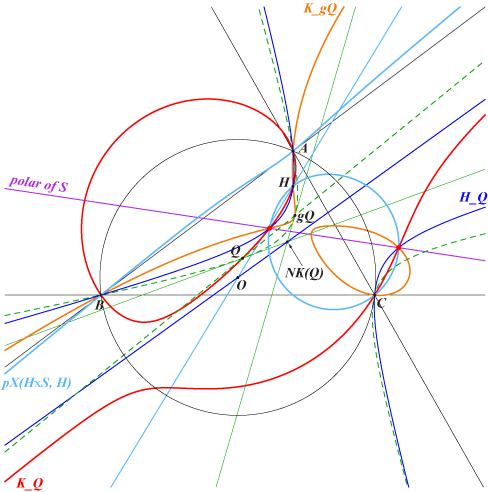Orthic line of a circular pK A circular pK(Ω, P) always has a proper orthic line (L) since it cannot be a stelloid. Recall that (L) is the locus of points whose polar conic in the pK is a rectangular hyperbola. It is also the Laplacian of the cubic. Note that (L) passes through the real infinite point of the circular pK. Special cases • when Ω = X(6), the cubic is an isogonal pK with pivot P on the line at infinity, and then (L) is the line OP. (L) also passes through O when P lies on the Jerabek hyperbola in which case Ω lies on K381 = cK(#X6, X523). • when P = H = X(4), the cubic is an isogonal pK with respect to the orthic triangle. See CL019. Its pole Ω lies on the orthic axis, its isopivot H* lies on the line at infinity, and then (L) is the line X(5)H*. (L) also passes through X(5) when Ω lies on the circum-conic with perspector X(512) in which case P lies on K025. *** When P is given, recall that Ω is the barycentric product P x igP and (L) is parallel to the line P, gcP. (L) passes through a given point Q ≠ O if and only if P lies on a circular circum-cubic K(Q) with singular focus F, passing through H and the infinite point of the line OQ. The orthic line of K(Q) is the parallel at X(5) to the line OQ. The mapping QF : Q → F is studied in Table 74. Some remarkable cases • when Q lies on K005, K(Q) is a focal cubic with singular focus F on K060. If QaQbQc is the pedal triangle of Q and TaTbTc its image under the homothety h(P, –2), then F is the perspector of ABC and TaTbTc. See K005, locus property 2. • when Q is on the line at infinity, K(Q) is the antigonal transform of psK(gQ, tQ, H) with singular focus on the circle C(H, R) passing through X(265). • when Q is on the Euler line, K(Q) passes through X(30), X(265) and its tangent at H passes through X(51) except for Q = X(5) since the cubic is the nodal cubic K025 with node H. The singular focus lies on the line X(4), X(265) and the orthic line is the Euler line itself except K(O) which decomposes into the line at infinity and the Jerabek hyperbola. See green lines in tables below. • when Q is on the Brocard axis, K(Q) passes through X(511), X(32618), X(32619). The singular focus lies on the Fermat axis and the orthic line is parallel at X(5) to the Brocard axis. See yellow lines in tables below. *** Generalization Let (L) be a line passing through O, with infinite point Q whose isogonal conjugate is denoted Q*.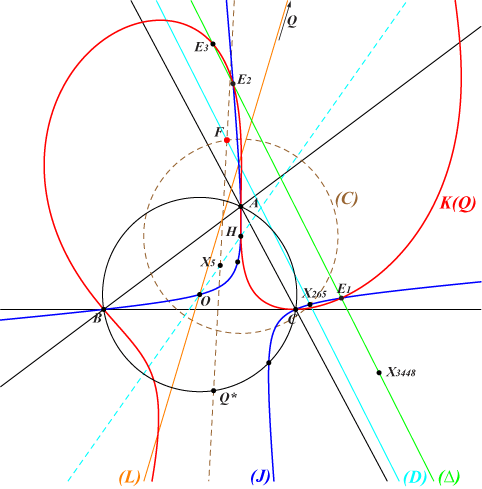If M is a variable point on the line (L), the cubics K(M) belong to a same pencil of circular circum-cubics passing through H, Q and two remaining points E1, E2 which lie on the Jerabek hyperbola (J) and on a line (∆) defined below. This pencil contains K(O) which is the union of the line at infinity and (J). It also contains K(Q), the antigonal transform of psK(gQ, tQ, H) with singular focus F on the circle (C) with center H, radius R, which passes through X(265). F is the reflection of Q* in X(5). The singular focus of every cubic K(M) lies on the line (D) passing through X(265) and F. (∆) is the image of (D) under the homothety h(H, 2). (∆) passes through X(3448) and meets K(Q) at a third point E3, the reflection of H in F. *** For a given line (L), the pencil K(M) also contains : • a K0 obtained when M is on the line GK. • two focal cubics obtained when M ≠ O is on the Napoleon cubic K005. • three psKs obtained when M is on a cubic (K1) passing through X(2), X(6),X(524) and X(182), corresponding to the three pKs K060, K337, K008 and K1134 respectively. X(15462) is another point on (K1). • two nKs obtained when M ≠ O is on a cubic (K2) passing through X(3), X(1147) – giving two decomposed cubics – and X(54) – giving the focal cubic K1154. Note that these different cubics are not necessarily all distinct nor real. *** In the tables below, the grey lines show the cubics obtained when (L) passes through X(54). All the cubics K(M) pass through X(3), X(33565) on (J) and X(1154) on the line at infinity.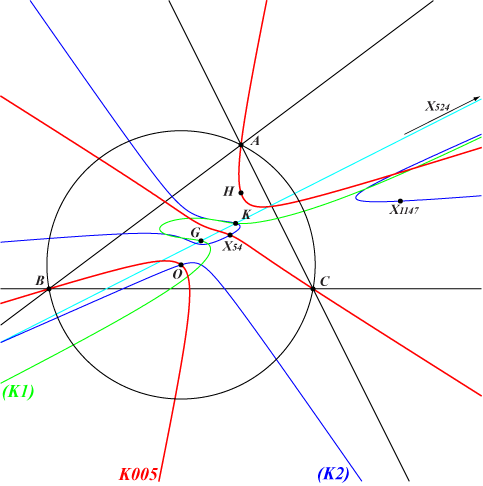The following tables show a good selection of cubics K(Q) obtained with the friendly help of Peter Moses. See additional notes for the coloured cells below the three tables. Listed cubicsQ K(Q) X(i) on K(Q) for i = 1 K529 1, 4, 80, 355, 517, 5903, 14987, 33599 2 K060 4, 5, 13, 14, 30, 79, 80, 265, 621, 622, 1117, 1141, 5627, 6761, 11581, 11582, 11584, 11600, 11601, 14372, 14373, 14451, 14452, 17405, 17406, 19658, 33664, 34295, 34296, 34297, 34298, 34299, 34300, 34301, 34302, 34303, 34304, 34305 4 K530 4, 30, 265, 477, 7728, 14989 5 K025 4, 30, 265, 316, 671, 1263, 1300, 5080, 5134, 5203, 5523, 5962, 10152, 11604, 11605, 11703, 13495, 16172, 19552, 31862, 31863, 34150, 34169, 34170, 34171, 34172, 34173, 34174, 34175, 34239, 34240, 37888 6 K337 4, 114, 371, 372, 511, 2009, 2010, 3563, 5000, 5001, 32618, 32619 30 K449 4, 20, 30, 146, 265, 1138, 1294 54 K1154 3, 4, 1141, 1154, 2070, 25043, 25044, 33565 61 K1132b 4, 14, 15, 62, 511, 621, 1337, 5617, 11601, 32618, 32619, 34219 62 K1132a 4, 13, 16, 61, 511, 622, 1338, 5613, 11600, 32618, 32619, 34220 182 K1134 4, 76, 98, 511, 1342, 1343, 5004, 5005, 9469, 11610, 15407, 32618, 32619, 34237, 34238, 34239, 34240 195 K465 3, 4, 5, 1154, 1157, 1263, 14072, 14979, 14980, 19552, 24772, 33565 381 K427 4, 30, 265, 382, 1539, 20480, 33641 511 K1131 4, 147, 315, 511, 1297, 1670, 1671, 1916, 5002, 5003, 32618, 32619, 34137, 34214 524 K008 2, 4, 67, 69, 316, 524, 671, 858, 2373, 11061, 13574, 14360, 14364, 34163, 34164, 34165, 34166 576 K305 4, 262, 316, 511, 671, 842, 6054, 9970, 32618, 32619, 34235, 34241Focal cubics (Q on K005, singular focus on K060)Q K(Q) X(i) on K(Q) for i = 1 K529 1, 4, 80, 355, 517, 5903, 14987, 33599 4 K530 4, 30, 265, 477, 7728, 14989 5 K025 4, 30, 265, 316, 671, 1263, 1300, 5080, 5134, 5203, 5523, 5962, 10152, 11604, 11605, 11703, 13495, 16172, 19552, 31862, 31863, 34150, 34169, 34170, 34171, 34172, 34173, 34174, 34175, 34239, 34240, 37888 17 4, 13, 622, 11600, 11602, 20428 18 4, 14, 621, 11601, 11603, 20429 54 K1154 3, 4, 1141, 1154, 2070, 25043, 25044, 33565 61 K1132b 4, 14, 15, 62, 511, 621, 1337, 5617, 11601, 32618, 32619, 34219 62 K1132a 4, 13, 16, 61, 511, 622, 1338, 5613, 11600, 32618, 32619, 34220 195 K465 3, 4, 5, 1154, 1157, 1263, 14072, 14979, 14980, 19552, 24772, 33565 627 4, 13, 17, 622, 633, 3479, 8174, 11600, 34219, 36766 628 4, 14, 18, 621, 634, 3480, 8175, 11601, 34220 2120 4, 3481, 34304 2121 4, 3482 3336 4, 79, 484, 517, 5902, 13143 3459 4, 1263, 11584, 19552, 24144, 25148, 31392 3460 4, 7165, 34300 3461 4, 3483 3462 4, 6761, 8439 3463 4, 3484, 33664 3467 4, 3065, 19658 3468 4, 3466, 34301 3469 4, 3465, 34303 3470 4, 399, 5627, 5663, 33567 3471 4, 1117, 1138, 3448 3489 4, 1337 3490 4, 1338 6191 4, 7059 6192 4, 7060 7344 4, 1276, 17405 7345 4, 1277, 17406 8837 4, 1337, 8175, 8471 8839 4, 1338, 8174, 8479 8918 4, 8462 8919 4, 8452 8929 4, 8172, 11581 8930 4, 8173, 11582Unlisted cubics through at least six ETC centersQ K(Q) X(i) on K(Q) for i = 15 4, 511, 633, 3389, 3390, 22507, 32618, 32619 16 4, 511, 634, 3364, 3365, 22509, 32618, 32619 17 focal 4, 13, 622, 11600, 11602, 20428 18 focal 4, 14, 621, 11601, 11603, 20429 21 1, 4, 30, 265, 3065, 6914 32 4, 511, 1687, 1688, 2698, 12177, 32618, 32619 39 4, 511, 1352, 1689, 1690, 32618, 32619 58 1, 4, 511, 5018, 10446, 32618, 32619 140 2, 4, 30, 98, 265, 3448, 12079 283 1, 4, 68, 74, 3465, 13754 371 4, 511, 637, 3385, 3386, 6231, 32618, 32619 372 4, 511, 638, 3371, 3372, 6230, 32618, 32619 386 4, 80, 256, 511, 32618, 32619 389 4, 186, 317, 511, 1299, 32618, 32619 517 4, 153, 517, 1295, 1320, 3436 519 4, 8, 80, 519, 2370, 5176, 21290 527 4, 7, 329, 527, 3254, 5057 532 4, 13, 532, 616, 622, 11122, 11600, 22649 533 4, 14, 533, 617, 621, 11121, 11601, 22648 549 4, 8, 30, 265, 631, 12317 569 4, 311, 511, 1141, 32618, 32619 575 2, 4, 23, 111, 263, 511, 11185, 32618, 32619 577 4, 511, 1298, 5562, 32618, 32619 578 4, 250, 264, 511, 1300, 5962, 15463, 32618, 32619 627 focal 4, 13, 17, 622, 633, 3479, 8174, 11600, 34219, 36766 628 focal 4, 14, 18, 621, 634, 3480, 8175, 11601, 34220 758 1, 4, 758, 3869, 5080, 11604 970 4, 386, 511, 573, 11609, 32618, 32619 1125 4, 7, 80, 516, 4295, 5816 1154 3, 4, 1154, 3153, 18401, 33565 1385 4, 8, 104, 517, 12247, 34242 1493 3, 4, 1154, 2383, 33565, 37943 1503 4, 1503, 12384, 34168, 34239, 34240 1656 4, 30, 115, 265, 381, 10113, 13530, 33601 1790 4, 68, 74, 1276, 1277, 13754 1994 4, 15, 16, 1263, 6243, 16837, 19552 2055 4, 511, 577, 578, 3484, 32618, 32619 2092 4, 511, 1685, 1686, 32618, 32619 2393 4, 23, 66, 895, 1370, 2393 2888 4, 1141, 1263, 6288, 19552, 32423 3094 4, 511, 3102, 3103, 32618, 32619 3095 4, 39, 511, 5207, 32618, 32619, 37841 3098 4, 511, 7768, 29011, 32618, 32619 3336 focal 4, 79, 484, 517, 5902, 13143 3357 4, 2693, 6000, 10152, 15318, 34170 3368 4, 511, 3379, 3380, 32618, 32619 3379 4, 511, 3395, 3396, 32618, 32619 3393 4, 511, 3368, 3369, 32618, 32619 3395 4, 511, 3393, 3394, 32618, 32619 3398 4, 32, 182, 511, 18906, 32618, 32619 3459 focal 4, 1263, 11584, 19552, 24144, 25148, 31392 5012 3, 4, 1154, 3438, 3439, 5899, 13597, 33565 5019 4, 511, 10441, 13332, 13333, 32618, 32619 5092 4, 511, 5984, 29180, 32618, 32619 5398 4, 511, 953, 17139, 32618, 32619 5476 4, 316, 671, 14492, 19924, 31670, 32271 5694 4, 104, 2771, 5080, 5887, 11604 5889 3, 4, 1154, 18403, 22751, 33565 5893 4, 20, 10152, 11744, 13219, 15077, 34170 6102 3, 4, 562, 1154, 32710, 33565 6199 4, 511, 1151, 3312, 32618, 32619 6395 4, 511, 1152, 3311, 32618, 32619 6425 4, 511, 6200, 6420, 32618, 32619 6426 4, 511, 6396, 6419, 32618, 32619 6642 4, 25, 30, 265, 1112, 3563 6644 4, 24, 30, 265, 1299, 1986 6696 4, 253, 1503, 10152, 12324, 13573, 34170 6759 4, 1294, 6000, 14249, 34239, 34240 7617 4, 316, 671, 17503, 32479, 33601 7751 4, 76, 316, 538, 671, 2367 7758 4, 67, 69, 141, 524, 5207, 33665 7759 4, 83, 315, 316, 671, 754, 6328 7764 2, 4, 754, 2857, 11606, 20022, 36163, 37841 8542 4, 66, 111, 316, 671, 895, 2393, 10422, 11188 8546 4, 23, 67, 69, 524, 5354, 10630 8548 4, 25, 111, 858, 2987, 14984 8550 4, 67, 69, 98, 524, 5485, 7735, 16092 9019 4, 6, 22, 5189, 9019, 18125 9690 4, 511, 6409, 6418, 32618, 32619 9737 4, 511, 2710, 9289, 32618, 32619 10282 4, 20, 23, 1297, 6000, 32319 10627 3, 4, 1154, 5189, 16030, 29011, 33565 11126 3, 4, 13, 15, 622, 1154, 5616, 11600, 33565, 36981 11127 3, 4, 14, 16, 621, 1154, 5612, 11601, 33565, 36979 11165 4, 67, 69, 524, 2482, 15069, 15534, 33601 11178 4, 316, 671, 3818, 11645, 14458 11271 4, 1263, 1487, 3519, 5900, 19552 11438 4, 340, 511, 32618, 32619, 32710 12038 4, 20, 68, 74, 186, 13754, 15469 12161 3, 4, 403, 1154, 1299, 8883, 14111, 33565 13323 4, 58, 104, 314, 511, 572, 32618, 32619 13335 4, 511, 6776, 9292, 32618, 32619 13336 4, 511, 1232, 13597, 32618, 32619 13346 4, 511, 1294, 14615, 32618, 32619 13352 4, 477, 511, 3260, 32618, 32619 14520 4, 511, 991, 4253, 32618, 32619 14627 4, 511, 1263, 3447, 3613, 10263, 19552, 32618, 32619 15311 4, 3346, 6225, 10152, 15311, 34170 15345 3, 4, 195, 1154, 2888, 11584, 33565 15733 4, 1156, 3434, 6601, 15733, 17615 15774 4, 30, 265, 340, 2133, 5667 15781 4, 30, 265, 3484, 8431, 21659 16266 3, 4, 97, 858, 1154, 1297, 33565 17733 4, 80, 314, 740, 4647, 20558 22802 4, 1294, 2777, 5878, 10152, 34170 23329 4, 10152, 14216, 15319, 18400, 34170 32046 3, 4, 23, 98, 1154, 11140, 14652, 33565 34117 4, 1297, 2781, 5523, 11605, 19149 34507 4, 98, 316, 542, 671, 1352 34508 4, 14, 316, 531, 621, 671, 11601 34509 4, 13, 316, 530, 622, 671, 11600 35195 3, 4, 484, 1154, 3065, 33565 37814 4, 30, 186, 265, 7722, 32710Additional notes • when Q lies on the line X(2), X(17), X(62), X(532), X(627), etc, the cubics K(Q) are in a same pencil and pass through X(4), X(13), X(622), X(11600). Their singular foci lie on the circle through X(13), X(265), X(622), X(3448), X(5613), X(11600). This pencil contains three focal cubics, one being K1132a. See pink cells. • when Q lies on the line X(2), X(18), X(61), X(533), X(628), etc, the cubics K(Q) are in a same pencil and pass through X(4), X(14), X(621), X(11601). Their singular foci lie on the circle through X(14), X(265), X(621), X(3448), X(5617), X(11601). This pencil contains three focal cubics, one being K1132b. See orange cells. • when Q lies on the line X(5), X(524), X(576), etc, the cubics K(Q) are in a same pencil and pass through X(316), X(671) and X(4) counted twice since the tangent at X(4) is the same for all cubics, namely the line X(4)X(2393). Their singular foci lie on the circle through X(265), X(381), X(10748). This pencil contains three focal cubics, one being K025. See light blue cells. *** More generally, when Q lies on a line (L), the locus of singular foci of the cubics K(Q) is a circle passing through X(265), the focus of K025. If (L) passes through O, this circle splits into the line at infinity and a line passing through X(265). If (L) is the perpendicular bisector of OH, all the cubics K(Q) share the same singular focus, namely X(265).